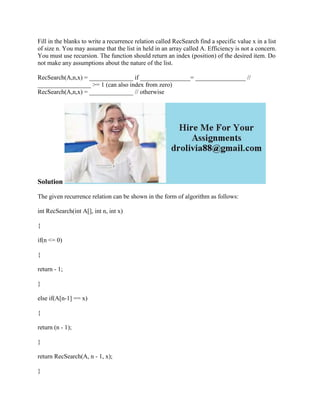Se está descargando tu SlideShare. ×

# Fill in the blanks to write a recurrence relation called RecSearch fin.docx

Anuncio
Anuncio
Anuncio
Anuncio
Anuncio
Anuncio
Anuncio
Anuncio
Anuncio
Anuncio
AnuncioCargando en…3
×

1 de 2 Anuncio

# Fill in the blanks to write a recurrence relation called RecSearch fin.docx

Fill in the blanks to write a recurrence relation called RecSearch find a specific value x in a list of size n. You may assume that the list in held in an array called A. Efficiency is not a concern. You must use recursion. The function should return an index (position) of the desired item. Do not make any assumptions about the nature of the list.
RecSearch(A,n,x) = ______________ if ________________= ________________ // _________________ >= 1 (can also index from zero)
RecSearch(A,n,x) = ______________ // otherwise
Solution
The given recurrence relation can be shown in the form of algorithm as follows:
int RecSearch(int A[], int n, int x)
{
if(n <= 0)
{
return - 1;
}
else if(A[n-1] == x)
{
return (n - 1);
}
return RecSearch(A, n - 1, x);
}
The recurrence relation RecSearch(A, n, x) will return -1 if the value of n is less than or equal to 0. The recurrence relation RecSearch(A, n, x) will return the index of the element if the condition if(A[n â€“ 1] == x) is satisfied.
The RecSearch(A, n, x) will return the index returned by the multiple calls to the RecSearch(A, n - 1, x).
The blanks given in the problem can be filled as follows:
RecSearch(A, n, x) = -1 if n < = 0 // if n >= 1 (can also index from zero) RecSearch(A, n, x) = RecSearch(A, n â€“ 1, x) // otherwise.
.

Fill in the blanks to write a recurrence relation called RecSearch find a specific value x in a list of size n. You may assume that the list in held in an array called A. Efficiency is not a concern. You must use recursion. The function should return an index (position) of the desired item. Do not make any assumptions about the nature of the list.
RecSearch(A,n,x) = ______________ if ________________= ________________ // _________________ >= 1 (can also index from zero)
RecSearch(A,n,x) = ______________ // otherwise
Solution
The given recurrence relation can be shown in the form of algorithm as follows:
int RecSearch(int A[], int n, int x)
{
if(n <= 0)
{
return - 1;
}
else if(A[n-1] == x)
{
return (n - 1);
}
return RecSearch(A, n - 1, x);
}
The recurrence relation RecSearch(A, n, x) will return -1 if the value of n is less than or equal to 0. The recurrence relation RecSearch(A, n, x) will return the index of the element if the condition if(A[n â€“ 1] == x) is satisfied.
The RecSearch(A, n, x) will return the index returned by the multiple calls to the RecSearch(A, n - 1, x).
The blanks given in the problem can be filled as follows:
RecSearch(A, n, x) = -1 if n < = 0 // if n >= 1 (can also index from zero) RecSearch(A, n, x) = RecSearch(A, n â€“ 1, x) // otherwise.
.

Anuncio
Anuncio

### Fill in the blanks to write a recurrence relation called RecSearch fin.docx

1. 1. Fill in the blanks to write a recurrence relation called RecSearch find a specific value x in a list of size n. You may assume that the list in held in an array called A. Efficiency is not a concern. You must use recursion. The function should return an index (position) of the desired item. Do not make any assumptions about the nature of the list. RecSearch(A,n,x) = ______________ if ________________= ________________ // _________________ >= 1 (can also index from zero) RecSearch(A,n,x) = ______________ // otherwise Solution The given recurrence relation can be shown in the form of algorithm as follows: int RecSearch(int A[], int n, int x) { if(n <= 0) { return - 1; } else if(A[n-1] == x) { return (n - 1); } return RecSearch(A, n - 1, x); }
2. 2. The recurrence relation RecSearch(A, n, x) will return -1 if the value of n is less than or equal to 0. The recurrence relation RecSearch(A, n, x) will return the index of the element if the condition if(A[n â€“ 1] == x) is satisfied. The RecSearch(A, n, x) will return the index returned by the multiple calls to the RecSearch(A, n - 1, x). The blanks given in the problem can be filled as follows: RecSearch(A, n, x) = -1 if n < = 0 // if n >= 1 (can also index from zero) RecSearch(A, n, x) = RecSearch(A, n â€“ 1, x) // otherwise.